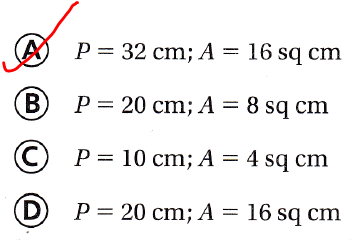# Texas Go Math Grade 5 Lesson 11.4 Answer Key Properties of Two-Dimensional Figures

Refer to our Texas Go Math Grade 5 Answer Key Pdf to score good marks in the exams. Test yourself by practicing the problems from Texas Go Math Grade 5 Lesson 11.4 Answer Key Properties of Two-Dimensional Figures.

## Texas Go Math Grade 5 Lesson 11.4 Answer Key Properties of Two-Dimensional Figures

Essential Question
How can you use the strategy  “Act it out” to approximate whether the sides of a figure are congruent?
The strategy to find whether the sides of a figure are congruent or not is:
Step 1:
Draw a figure with the given specifications
Step 2:
Measure the side lengths
Step 3:
If any 2 of the side lengths are the same, then those 2 lines are congruent
Step 4:
Repeat step 3 to check whether there are other side lengths that are the same

Unlock the problem

Lori has a quadrilateral with vertices A, B, C, and D. The quadrilateral has four right angles. She wants to show that quadrilateral ABCD is a square, but she does not have a ruler to measure the lengths of the sides. How can she show that the quadrilateral has four congruent sides and is a square?What do I need to find?
I need to determine whether the quadrilateral has 4 congruent sides and is a square

What information am I given?

The quadrilateral has 4 right angles. To be a square, it must also have 4 congruent sides.

Plan

What is my plan or strategy?
I can trace the figure, cut it out, and then fold it to match each pair of sides to show that sides are equal and congruent

Solve

I traced the quadrilateral and cut it out. I used  “Act it out” by folding to match each pair of sides.

• 1 folded the quadrilateral to match side AB to side DC.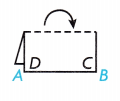• I folded the quadrilateral to match side AD to side BC.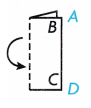• 1 folded the quadrilateral A diagonally to match side AD to side AB and side CD to side CB.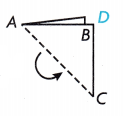Question 1.
What else do you need to do to solve the problem?
You need the length of 4 sides of the given quadrilateral ABCD to solve the given problem
So,
The quadrilateral ABCD is a square.

Try Another Problem

Terrence has drawn a triangle with vertices E, F, and G. The triangle has three congruent angles. He wants to show that the triangle EFG has three congruent sides, but he does not have a ruler to measure the lengths of the sides. How can he show that the triangle has three congruent sides?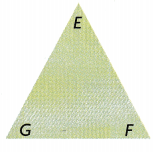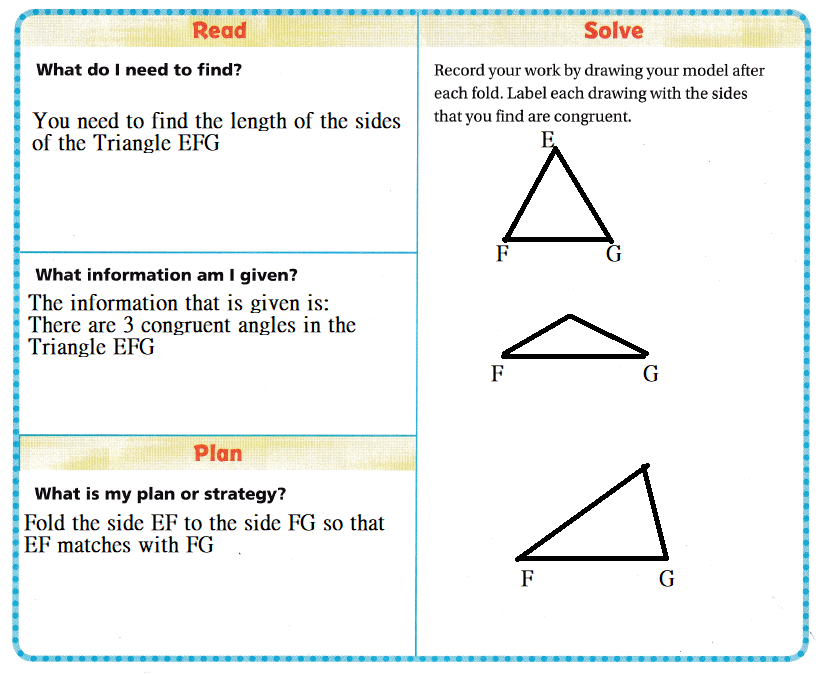How can you use reasoning to show that all three sides of the triangle are congruent using just two folds? Explain.
When we folded the triangle EFG,
If the sides are matched with each other, then we can say that the sides are congruent
Hence, from the above,
We can conclude that
We can show that all three sides of the triangle are congruent by using just two folds

Share and Show

Question 1.
Erica thinks that triangle XYZ, at the right, has two congruent sides, but she does not have a ruler to measure the sides. Are two sides congruent?
First, trace the triangle and cut out the tracing.
Then, fold the triangle to match each pair of sides to determine if at least two of the sides are congruent. As you test the sides, record or draw the results for each pair to make sure that you have checked all pairs of sides.It is given that
Erica thinks that triangle XYZ, at the right, has two congruent sides, but she does not have a ruler to measure the sides
Now,
Step 1:
Draw a triangle XYZ with some lengths and with congruent angles
Step 2:
Cut the line segment XY and fold the remaining portion
Step 3:
The cut portion and the folded portion matches perfectly. So, the 2 line segments are congruent
Hence, from the above,
We can conclude that
The two sides are congruent

Math Talk
Mathematical Processes
What if Erica also wants to show, without using a protractor, that the triangle has one right angle and two acute angles? Explain how she can show this.
In the Triangle XYZ, if the line is perpendicular, then
We know that the perpendicular line has the angle of 90° and the remaining two line segments are not perpendicular lines and hence the two line segments have the acute angles

Question 2.
December, January, and February were the coldest months in Kristen’s town last year. February was the warmest of these months. December was not the coldest. What is the order of these months from coldest to warmest?
It is given that
December, January, and February were the coldest months in Kristen’s town last year. February was the warmest of these months. December was not the coldest
So,
The order of the given months from the coldest to warmest is:
January > December > February
Hence, from the above,
We can conclude that
The order of the given months from the coldest to warmest is:
January > December > February

Problem Solving

H.O.T. Multi-Step Jan enters a 20-foot by 30-foot rectangular room. The long sides face north and south. Jan enters the exact center of the south side and walks 10 feet north. Then she walks 8 feet east. How far is she from the east side of the room?
It is given that
Jan enters a 20-foot by 30-foot rectangular room. The long sides face north and south. Jan enters the exact center of the south side and walks 10 feet north. Then she walks 8 feet east
Now,
According to the given information,
The distance of Jan from the east side of the room = (30 – 15 + 10) – 8
= 17 feet
Hence, from the above,
We can conclude that
The distance of Jan from the east side of the room is: 17 feet

Question 4.
Use Diagrams Max drew a grid to divide a piece of paper into 18 congruent squares. What is the least number of lines Max can draw to divide the grid into 6 congruent rectangles? Use the diagram to show your reasoning.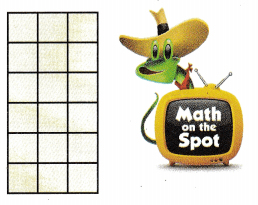It is given that
Max drew a grid to divide a piece of paper into 18 congruent squares
Now,
The given grid is:Now,
According to the given information,
The least number of lines Max can draw to divide the grid into 6 congruent rectangles = $$\frac{18}{6}$$
= 3 lines
Hence, from the above,
We can conclude that
The least number of lines Max can draw to divide the grid into 6 congruent rectangles is: 3 lines

Question 5.
Pierre uses a sheet of printer paper for origami. How can he check if the paper is a rectangle?
(A) Use a square corner to see if the paper has four right angles.
(B) Fold the paper in half both ways to see if the opposite sides are congruent.
(C) Both A and B
(D) Neither A nor B
It is given that
Pierre uses a sheet of printer paper for origami.
Hence, from the above,
We can conclude that
The steps to check whether the given paper is a rectangle or not is: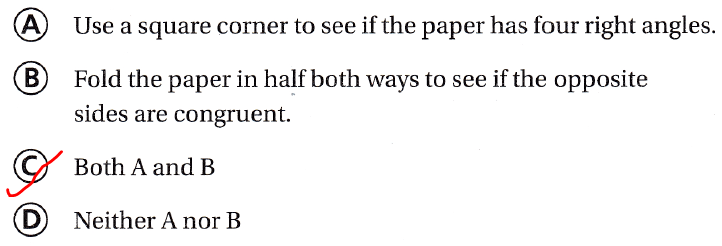Use Math Language Meaghan has drawn and cut out triangle LMN. She folds side LM to side MN and they match evenly. She folds side MN to side NL and they do not match evenly. Which of these describes Meaghan’s triangle?
(A) scalene triangle
(B) equilateral triangle
(C) isosceles triangle
(D) right triangle
It is given that
Meaghan has drawn and cut out triangle LMN. She folds side LM to side MN and they match evenly. She folds side MN to side NL and they do not match evenly
Hence, from the above,
We can conclude that
The type of triangle that describes Meaghan’s triangle is: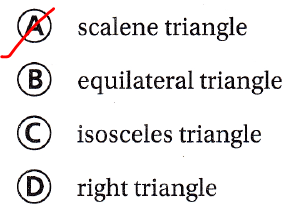Question 7.
Multi-Step Bethany uses six congruent equilateral triangles to create a polygon. Which of the following describes the polygon she creates?
(A) regular pentagon
(B) regular hexagon
(C) square
(D) regular triangle
It is given that
Bethany uses six congruent equilateral triangles to create a polygon
Hence, from the above,
We can conclude that
The type of polygon that describes the polygon Bethany created is:Texas Test Prep

Which figure below is a quadrilateral that has opposite sides that are congruent and parallel?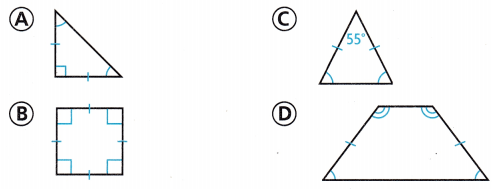The quadrilateral that have opposite sides that are congruent and parallel are: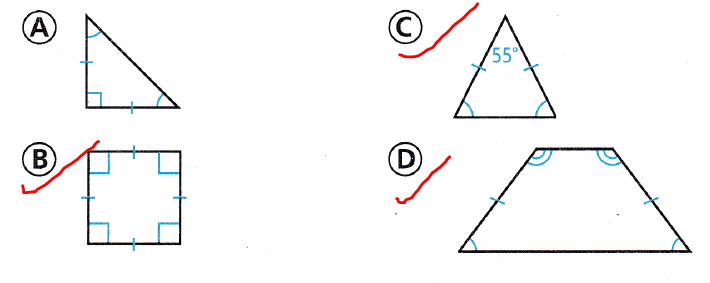### Texas Go Math Grade 5 Lesson 11.4 Homework and Practice Answer Key

Question 1.
Explain a strategy you can use to draw a figure that is congruent to the figure at the right.The given figure is:Now,
The strategy that you can use to draw a figure that is congruent to the given figure is:
Step 1:
Measure the lengths of the line segments of the given figure
Step 2:
Reproduce the lengths of the line segments of the given figure to the right of the given figure
Step 3:
Represent the angles that is present to the right side of the given figure so that the given figure’s angle measures and the figure that is to the right’s angle measures are congruent

Go Math Grade 5 Practice and Homework Lesson 11.4 Answer Key Question 2.
Classify the figure at the right in as many ways as possible. Explain your strategy.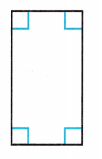The given figure is:Now,
From the given figure,
We can observe that
The parallel sides are congruent and equal
The angle measures are 90° i..e., congruent angle measures
Hence, from the above,
We can conclude that
The given figure is: Rectangle

Question 3.
Draw two polygons with the same number of sides and angles in each polygon. Make one of the polygons regular and one of the polygons not regular. Are the figures congruent? Explain.
It is given that
Draw two polygons with the same number of sides and angles in each polygon. Make one of the polygons regular and one of the polygons not regular
Now,
According to the given information,
In a Regular polygon, all the side lengths and angle measures are congruent
In an irregular polygon, all the side lengths and angle measures are not congruent
Hence, from the above,
We can conclude that
The two polygons are not congruent

Question 4.
Combine the two congruent trapezoids at the right to make new polygons. Draw your new polygons and classify them.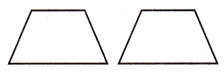The given figure is:Hence, from the above,
We can conclude that
The combination of two congruent trapezoids is: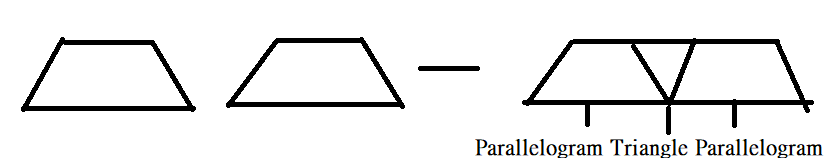Problem Solving

Shoshanna draws a square. What polygons can she make if she draws a line that cuts the square in half? Explain.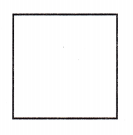It is given that
Shoshanna draws a square
Now,
The given figure is:Now,
When we cut the given figure into two halves,
The two squares will be formed
Hence, from the above,
We can conclude that
The polygons that Shoshanna can make if she draws a line that cuts the square in half are: Squares

Lesson check

Texas Test Prep

Question 6.
A regular octagon has a total perimeter of 24 inches. What is the length of the octagon?
(A) 8 inches
(B) 1 inch
(C) 4 inches
(D) 3 inches
It is given that
A regular octagon has a total perimeter of 24 inches
Now,
We know that,
An “Octagon” has 8 sides
The “Perimeter” is the sum of all the side lengths
So,
The length of each side in the Octagon = $$\frac{Perimeter}{8}$$
= $$\frac{24}{8}$$
= 3 inches
Hence, from the above,
We can conclude that
The length of each side in the Octagon is: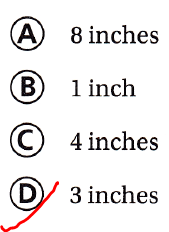Question 7.
Adolfo drew a quadrilateral. He says at least one side of one of the angles is acute. Which polygon could Adolfo have drawn?
(A) parallelogram
(B) square
(C) rectangle
(D) isosceles triangle
It is given that
Adolfo drew a quadrilateral. He says at least one side of one of the angles is acute
Now,
We know that,
Hence, from the above,
We can conclude that
The polygon that Adolfo can draw is: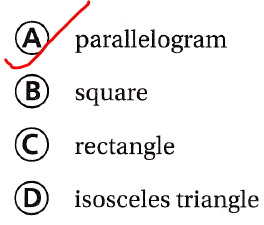Question 8.
Classify the polygon named MNPR in the figure below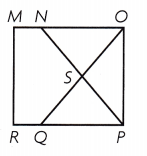(A) trapezoid
(B) triangle
(C) rectangle
(D) parallelogram
The given figure is:Hence, from the above,
We can conclude that
The polygon named MNPR is called as: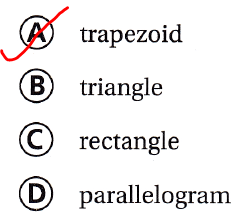(A) 2 pairs of parallel sides
(B) 2 pairs of perpendicular sides
(C) 1 pair of parallel sides
(D) no pairs of parallel sides
The given figure is:Now,
From the given figure,
We can observe that
The given figure is: Trapezoid
Hence, from the above,
We can conclude that
The options that describe the given quadrilateral is:Question 10.
Multi-Step Veronica folded an equilateral triangle in half and cut along the fold to make two congruent figures. What figures did she make?
(A) two scalene right triangles
(B) a triangle and a trapezoid
(C) two isosceles acute triangles
(D) two equilateral triangles
It is given that
Veronica folded an equilateral triangle in half and cut along the fold to make two congruent figures
Hence, from the above,
We can conclude that
The figures that Veronica can make is: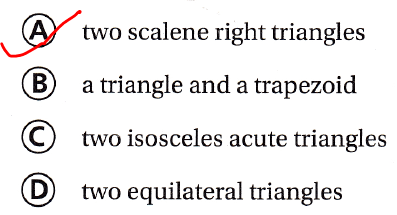Question 11.
Multi-Step Rocco places four congruent squares side by side in a row. The length of one side of a square ¡s 2 cm. What are the perimeter and the area of the larger rectangle Rocco makes?
(A) P = 32 cm; A = 16 sq cm
(B) P = 20 cm; A = 8 sq cm
(C) P = 10 cm; A = 4 sq cm
(D) P = 20 cm; A = 16 sq cm